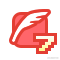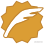# es6 import()函数_JavaScript_歪脖先生的博客-CSDN博客

## import()函数

### 简介

``复制代码``
1. `// 报错`
2. `if (x === 2) {`
3. ` import MyModual from './myModual';`
4. `}`

``复制代码``
1. `const path = './' + fileName;`
2. `const myModual = require(path);`

``复制代码``
1. `import(specifier)`

```ES6 import()```返回一个 Promise 对象。下面是一个例子。

``复制代码``
1. `const main = document.querySelector('main');`
2. `import(`./section-modules/\${someVariable}.js`)`
3. ` .then(module => {`
4. ` module.loadPageInto(main);`
5. ` })`
6. ` .catch(err => {`
7. ` main.textContent = err.message;`
8. ` });`

`import()`函数可以用在任何地方，不仅仅是模块，非模块的脚本也可以使用。它是运行时执行，也就是说，什么时候运行到这一句，也会加载指定的模块。另外，`import()`函数与所加载的模块没有静态连接关系，这点也是与`import`语句不相同。

`import()`类似于 Node 的`require`方法，区别主要是前者是异步加载，后者是同步加载。

### 适用场合

（1）按需加载。

`import()`可以在需要的时候，再加载某个模块。

``复制代码``
1. `button.addEventListener('click', event => {`
2. ` import('./dialogBox.js')`
3. ` .then(dialogBox => {`
4. ` dialogBox.open();`
5. ` })`
6. ` .catch(error => {`
7. ` /* Error handling */`
8. ` })`
9. `});`

（2）条件加载

`import()`可以放在`if`代码块，根据不同的情况，加载不同的模块。

``复制代码``
1. `if (condition) {`
2. ` import('moduleA').then(...);`
3. `} else {`
4. ` import('moduleB').then(...);`
5. `}`

（3）动态的模块路径

`import()`允许模块路径动态生成。

``复制代码``
1. `import(f())`
2. `.then(...);`

### 注意点

`import()`加载模块成功以后，这个模块会作为一个对象，当作`then`方法的参数。因此，可以使用对象解构赋值的语法，获取输出接口。

``复制代码``
1. `import('./myModule.js')`
2. `.then(({export1, export2}) => {`
3. ` // ...·`
4. `});`

``复制代码``
1. `import('./myModule.js')`
2. `.then(myModule => {`
3. ` console.log(myModule.default);`
4. `});`

``复制代码``
1. `import('./myModule.js')`
2. `.then(({default: theDefault}) => {`
3. ` console.log(theDefault);`
4. `});`

``复制代码``
1. `Promise.all([`
2. ` import('./module1.js'),`
3. ` import('./module2.js'),`
4. ` import('./module3.js'),`
5. `])`
6. `.then(([module1, module2, module3]) => {`
7. ` ···`
8. `});`

`import()`也可以用在 async 函数之中。

``复制代码``
1. `async function main() {`
2. ` const myModule = await import('./myModule.js');`
3. ` const {export1, export2} = await import('./myModule.js');`
4. ` const [module1, module2, module3] =`
5. ` await Promise.all([`
6. ` import('./module1.js'),`
7. ` import('./module2.js'),`
8. ` import('./module3.js'),`
9. ` ]);`
10. `}`
11. `main();`ixygj197875博客专家 原创文章 627获赞 274访问量 118万+ 关注 他的留言板